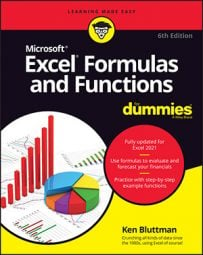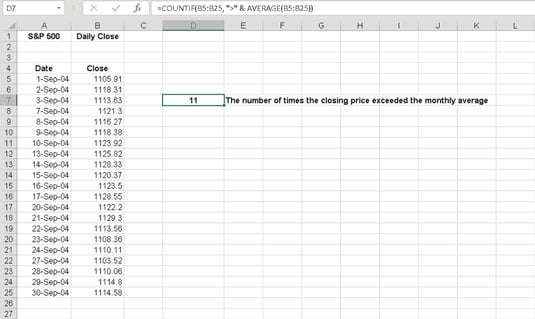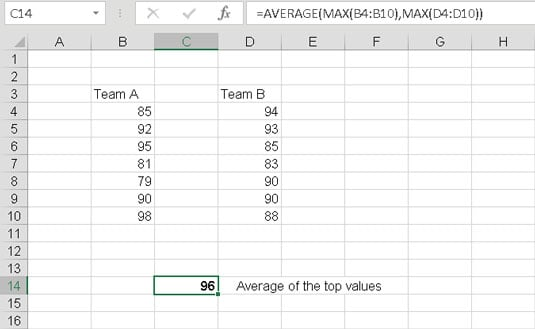##### Excel Formulas & Functions For DummiesA nested function is tucked inside another Excel function as one of its arguments. Nesting functions let you return results you would have a hard time getting otherwise.

The following figure shows the daily closing price for the Standard & Poor's 500 for the month of September 2004. A possible analysis is to see how many times the closing price was higher than the average for the month. Therefore, you need to calculate the average before you can compare any single price. Embed the AVERAGE function inside another function to calculate the average first.When a function is nested inside another, the inner function is calculated first. Then that result is used as an argument for the outer function.

The COUNTIF function counts the number of cells in a range that meet a condition. The condition in this case is that any single value in the range is greater than (>) the average of the range. The formula in cell D7 is =COUNTIF(B5:B25, ">" & AVERAGE(B5:B25)). The AVERAGE function is evaluated first; then the COUNTIF function is evaluated, using the returned value from the nested function as an argument.

Nested functions are best entered directly. The Insert Function dialog box does not make it easy to enter a nested function. Try one. In this example, you use the AVERAGE function to find the average of the largest values from two sets of numbers. The nested function in this example is MAX. You enter the MAX function twice within the AVERAGE function. Follow these steps:

1. Enter a few different numbers in one column.

2. Enter a few different numbers in a different column.

3. Click an empty cell where you want the result to appear.

4. Type =AVERAGE( to start the function entry.

5. Type MAX(.

6. Click the first cell in the second set of numbers, press the mouse button, and drag over all the cells of the first set.

The address of this range enters into the MAX function.

7. Enter a closing parenthesis to end the first MAX function.

8. Enter a comma (,).

9. Once again, type MAX(.

10. Click the first cell in the second set of numbers, press the mouse button, and drag over all the cells of the second set.

The address of this range enters into the MAX function.

11. Enter a closing parenthesis to end the second MAX function.

12. Enter a ).

This ends the AVERAGE function.

13. Press Enter.

This figure shows the result of your nested function. Cell C14 has this formula: =AVERAGE(MAX(B4:B10),MAX(D4:D10)).Getting a result from nested functions.

When you use nested functions, the outer function is preceded with an equal sign (=) if it is the beginning of the formula. Any nested functions are not preceded with an equal sign.

You can nest functions up to 64 levels.